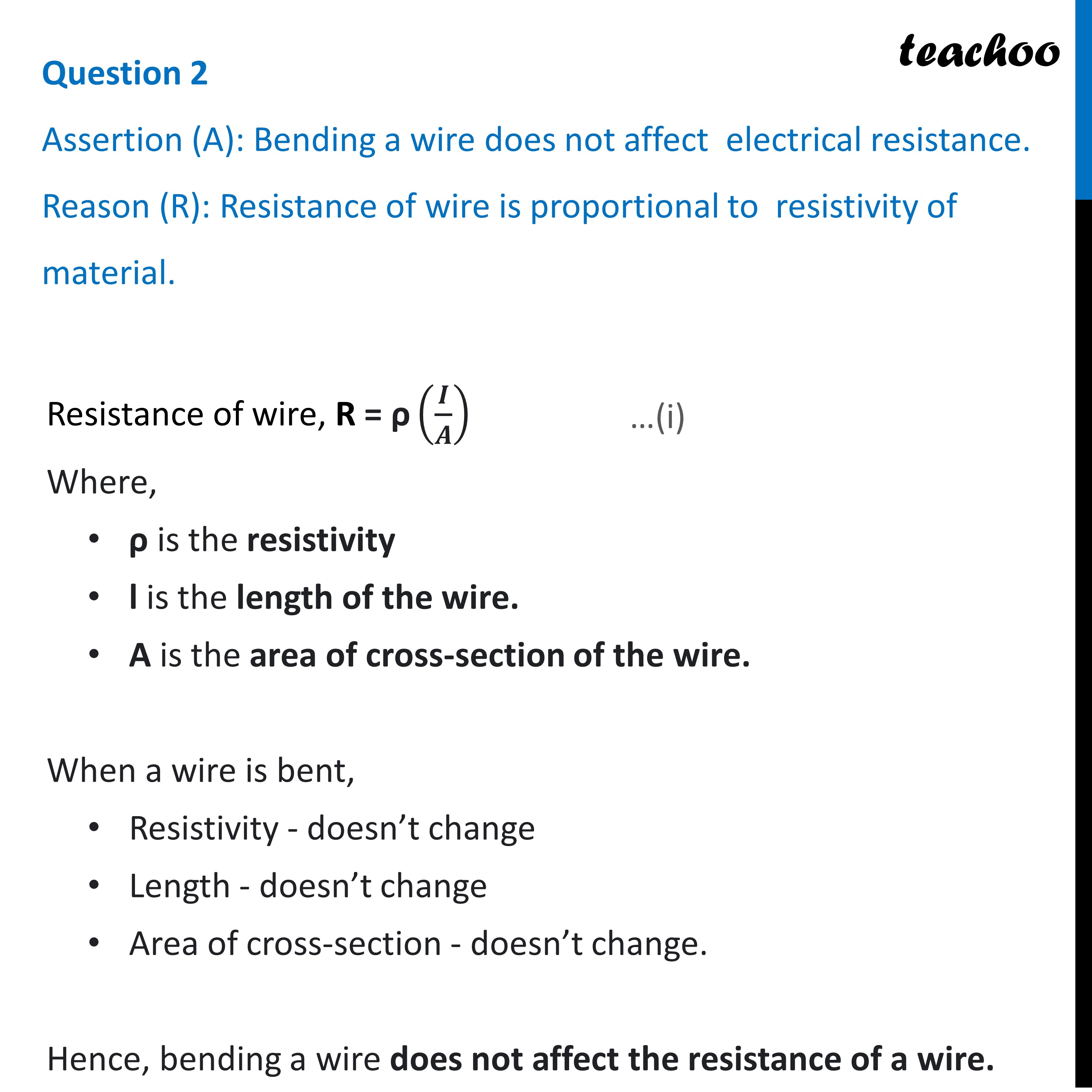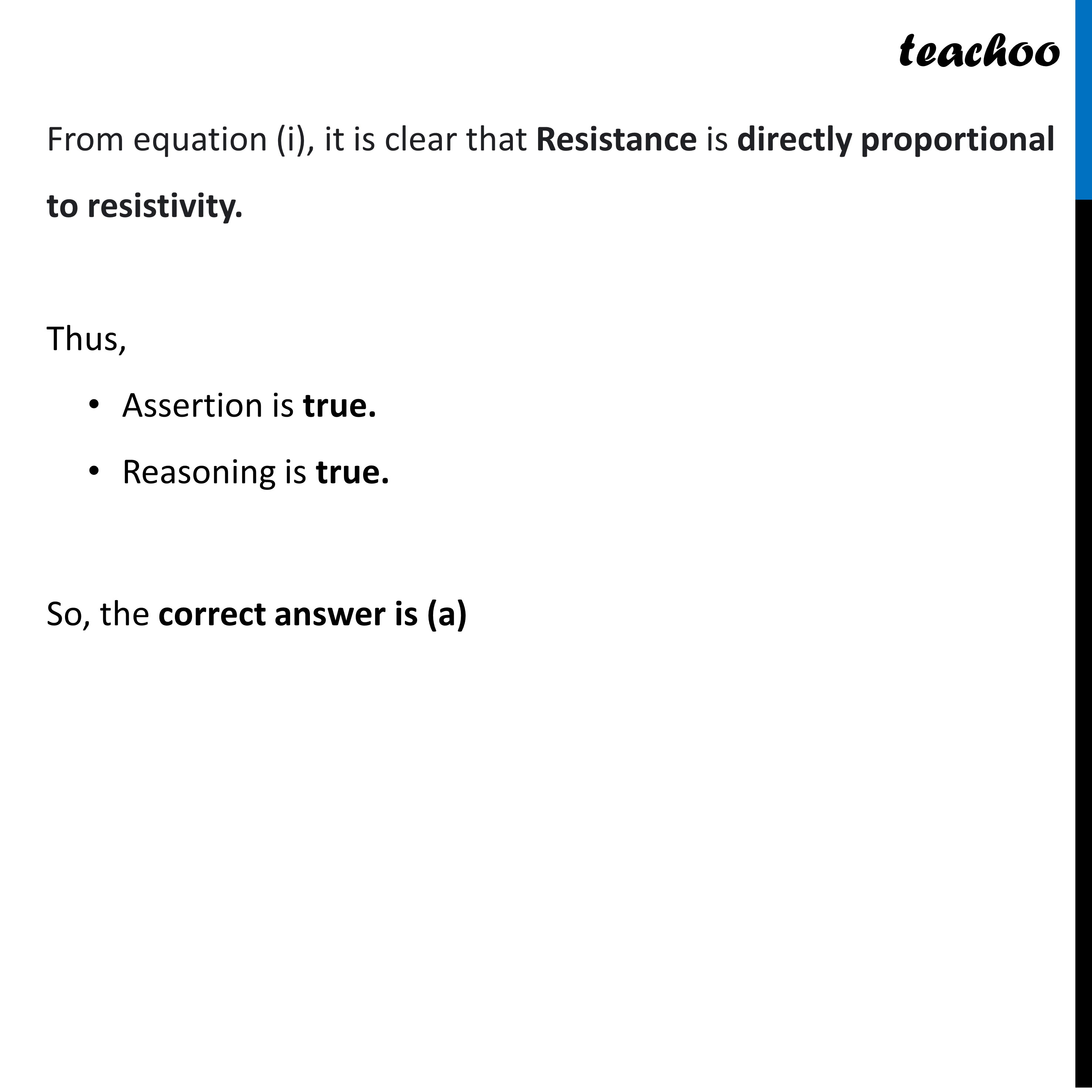Assertion Reasoning questions (MCQ)

Class 10
Chapter 12 Class 10 - Electricity

## Reason (R): Resistance of wire is proportional to resistivity of material.

Resistance of wire, R = ρ (l / A)        —----(i)

Where,

• ρ is the resistivity
• l is the length of the wire.
• A is the area of cross-section of the wire.

When a wire is bent,

• Resistivity - doesn’t change
• Length - doesn’t change
• Area of cross-section - doesn’t change.

Hence, bending a wire does not affect the resistance of a wire.

From equation (i), it is clear that Resistance is directly proportional to resistivity.

Thus,

• Assertion is true.
• Reasoning is true.

So, the correct answer is (a).Learn in your speed, with individual attention - Teachoo Maths 1-on-1 Class

### Transcript

Question 2 Assertion (A): Bending a wire does not affect electrical resistance. Reason (R): Resistance of wire is proportional to resistivity of material. Resistance of wire, R = "ρ" (𝑰/𝑨) Where, ρ is the resistivity l is the length of the wire. A is the area of cross-section of the wire. When a wire is bent, Resistivity - doesn’t change Length - doesn’t change Area of cross-section - doesn’t change. Hence, bending a wire does not affect the resistance of a wire.From equation (i), it is clear that Resistance is directly proportional to resistivity. Thus, Assertion is true. Reasoning is true. So, the correct answer is (a)